# Fewer than 500 sheep,

There are fewer than 500 sheep, but if they stand in a double, triple, quadruple, five, and sixth-order, one sheep will remain.
But they can stand in the seventh order. How many are sheep?

n =  301

### Step-by-step explanation:

a%b = a modulo b

n=7: n%2=1, n%3=1, n%4=3, n%5=2, n%6=1, n%7=0
n=14: n%2=0, n%3=2, n%4=2, n%5=4, n%6=2, n%7=0
n=21: n%2=1, n%3=0, n%4=1, n%5=1, n%6=3, n%7=0
n=28: n%2=0, n%3=1, n%4=0, n%5=3, n%6=4, n%7=0
n=35: n%2=1, n%3=2, n%4=3, n%5=0, n%6=5, n%7=0
n=42: n%2=0, n%3=0, n%4=2, n%5=2, n%6=0, n%7=0
n=49: n%2=1, n%3=1, n%4=1, n%5=4, n%6=1, n%7=0
n=56: n%2=0, n%3=2, n%4=0, n%5=1, n%6=2, n%7=0
n=63: n%2=1, n%3=0, n%4=3, n%5=3, n%6=3, n%7=0
n=70: n%2=0, n%3=1, n%4=2, n%5=0, n%6=4, n%7=0
n=77: n%2=1, n%3=2, n%4=1, n%5=2, n%6=5, n%7=0
n=84: n%2=0, n%3=0, n%4=0, n%5=4, n%6=0, n%7=0
n=91: n%2=1, n%3=1, n%4=3, n%5=1, n%6=1, n%7=0
n=98: n%2=0, n%3=2, n%4=2, n%5=3, n%6=2, n%7=0
n=105: n%2=1, n%3=0, n%4=1, n%5=0, n%6=3, n%7=0
n=112: n%2=0, n%3=1, n%4=0, n%5=2, n%6=4, n%7=0
n=119: n%2=1, n%3=2, n%4=3, n%5=4, n%6=5, n%7=0
n=126: n%2=0, n%3=0, n%4=2, n%5=1, n%6=0, n%7=0
n=133: n%2=1, n%3=1, n%4=1, n%5=3, n%6=1, n%7=0
n=140: n%2=0, n%3=2, n%4=0, n%5=0, n%6=2, n%7=0
n=147: n%2=1, n%3=0, n%4=3, n%5=2, n%6=3, n%7=0
n=154: n%2=0, n%3=1, n%4=2, n%5=4, n%6=4, n%7=0
n=161: n%2=1, n%3=2, n%4=1, n%5=1, n%6=5, n%7=0
n=168: n%2=0, n%3=0, n%4=0, n%5=3, n%6=0, n%7=0
n=175: n%2=1, n%3=1, n%4=3, n%5=0, n%6=1, n%7=0
n=182: n%2=0, n%3=2, n%4=2, n%5=2, n%6=2, n%7=0
n=189: n%2=1, n%3=0, n%4=1, n%5=4, n%6=3, n%7=0
n=196: n%2=0, n%3=1, n%4=0, n%5=1, n%6=4, n%7=0
n=203: n%2=1, n%3=2, n%4=3, n%5=3, n%6=5, n%7=0
n=210: n%2=0, n%3=0, n%4=2, n%5=0, n%6=0, n%7=0
n=217: n%2=1, n%3=1, n%4=1, n%5=2, n%6=1, n%7=0
n=224: n%2=0, n%3=2, n%4=0, n%5=4, n%6=2, n%7=0
n=231: n%2=1, n%3=0, n%4=3, n%5=1, n%6=3, n%7=0
n=238: n%2=0, n%3=1, n%4=2, n%5=3, n%6=4, n%7=0
n=245: n%2=1, n%3=2, n%4=1, n%5=0, n%6=5, n%7=0
n=252: n%2=0, n%3=0, n%4=0, n%5=2, n%6=0, n%7=0
n=259: n%2=1, n%3=1, n%4=3, n%5=4, n%6=1, n%7=0
n=266: n%2=0, n%3=2, n%4=2, n%5=1, n%6=2, n%7=0
n=273: n%2=1, n%3=0, n%4=1, n%5=3, n%6=3, n%7=0
n=280: n%2=0, n%3=1, n%4=0, n%5=0, n%6=4, n%7=0
n=287: n%2=1, n%3=2, n%4=3, n%5=2, n%6=5, n%7=0
n=294: n%2=0, n%3=0, n%4=2, n%5=4, n%6=0, n%7=0
n=301: n%2=1, n%3=1, n%4=1, n%5=1, n%6=1, n%7=0 <<<<<<=====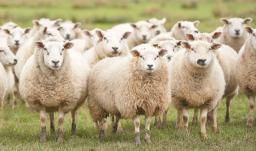Did you find an error or inaccuracy? Feel free to write us. Thank you!Tips to related online calculators
Do you want to calculate least common multiple two or more numbers?
Do you want to perform natural numbers division - find the quotient and remainder?

## Related math problems and questions:

• Sheep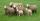Shepherd tending the sheep. Tourists asked him how much they have. The shepherd said, "there are fewer than 500. If I them lined up in 4-row 3 remain. If in 5-row 4 remain. If in 6-row 5 remain. But I can form 7-row." How many sheep have herdsman?
• Shepherd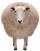The shepherd has fewer than 500 sheep; where they can be up to 2, 3, 4, 5, 6 row is always 1 remain, and as can be increased up to 7 rows of the sheep, and it is not increased no ovine. How many sheep has a shepherd?
• LCMWhat is the least common multiple of 5, 50, 14?On the meadow grazing horses, cows, and sheep, together with less than 200. If cows were 45 times more, horses 60 times more, and sheep 35 times more than there are now, their numbers would equally. How many horses, cows, and sheep are on the meadow toget
• Lcm simpleFind least common multiple of this two numbers: 140 175.
• Lcm of three numbersWhat is the Lcm of 120 15 and 5
• On Children'sOn Children's Day, the organizers bought 252 chewing gums, 396 candies and 108 lollipops. They want to make as many of the same packages as possible. Advise them what to put in each package and how many packages they can make this way.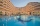Up to 48 rooms, some of which are triple and some quadruple, accommodated 173 people so that all beds are occupied. How many triple and how many quadruple rooms were there?
• Three Titanics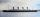Three steamers sailed from the same port on the same day. The first came back on the third day, fourth 4th day and the third returned sixth day. How many days after leaving the steamers met again in the harbor?
• 9.A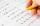9.A to attend more than 20 students but fewer than 40 students. A third of the pupils wrote a math test to mark 1, the sixth to mark 2, the ninth to mark 3. No one gets mark 4. How many students of class 9.A wrote a test to mark 5?
• LCM of two numberFind the smallest multiple of 63 and 147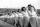Practitioners lined up in rectangle with row with four, five or six exercisers, one always missing to full rectangle. How many exercisers were on the field, if they have estimated not been more than 100?Winner can took win in three types of jettons with value 3, 30 and 100 dollars. What is minimal value of win payable in this values of jettons?In the bowl are plums. How many would be there if we can divide it equally among 8, 10 and 11 children?Less than 500 pupils attend school. When it is sorted into pairs, one pupil remains. Similarly, when sorted into 3, 4, 5 and 6 members team one remains. Sorted to seven members teams, no left behind. How many pupils are attending this school?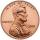Janko bought pencils for 35 cents each. Neither he nor the salesperson had small coins, just a whole € 1 coin. At least how many pencils had to buy to pay for the whole euros?Mom divided 24 apples and 15 pears to children. Each child received the same number of apples and pears - same number as his siblings. How many apples (j=?) and pears (h=?) received each child?